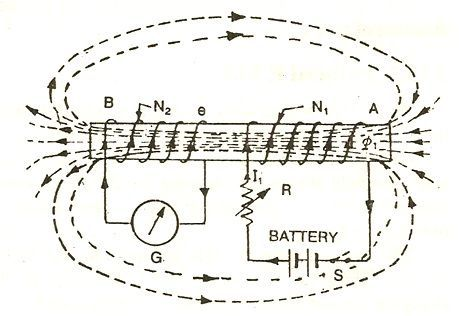# Mutually Induced EMF

Definition: The emf induced in a coil due to the change of flux produced by another neighbouring coil linking to it, is called Mutually Induced emf.  Let us take an example to understand the phenomenon of mutually induced emf.

Consider a coil AB. Coil B is having N2 number of turns and is placed near another coil A having N1 number of turns, as shown in the figure below:When the switch (S) is closed in the circuit shown above, current I1 flows through the coil A, and it produces the fluxφ1. Most of the flux says φ12 links with the other coil B.

If the current flowing through the coil A is changed by changing the value of variable resistor R, it changes flux linking with the other coil B and hence emf is induced in the coil. This induced emf is called Mutually Induced emf.

The direction of the induced emf is such that it opposes the cause which produces it, that means it opposes the change of current in the first coil. This effect of opposition caused by its own reason of production is called Lenz’s Law. A galvanometer (G) is connected to coil B for measuring the induced emf.

Since the rate of change of flux linking with the coil, B depends upon the rate of change of current in the coil A.The rate of change of current in coil A is directly proportional to the magnitude of the mutually induced emf.

M is called the Constant of Proportionality and is also called as Mutual Inductance or Coefficient of Mutual Inductance.